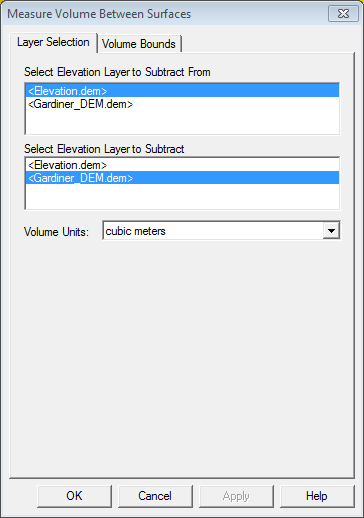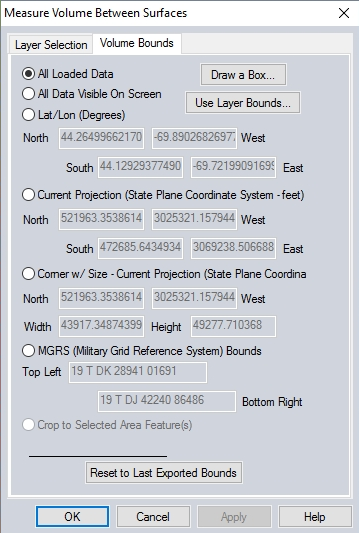# Calculating the Volume Between Two Surfaces

The commands to measure or calculate the volume between surfaces commands allow you to calculate the volume of material between two terrains or surfaces. Pile volume and cut and fill calculations are frequently used for determining the costs associated with moving material to or from a specific site, but may also be used for estimating agricultural yields, and determining the impact of a landslide event by comparing before and after volumetric calculations.

The Measure Volume Between Surfaces..., VOLUME - Measure Volume (Cut and Fill), and Pile Volume volumetric calculations work by dividing the area of interest up in to small rectangular pieces, and then calculating the sum volume of the small 3D rectangles between the two terrain models (Measure Volumes Between Surfaces) or the terrain model and the cut surface (Cut and Fill or Pile Volumes).

VOLUME - Measure Volume is found in the Analysis/Measurement Digitizer right-click menu and will measure the cut and fill volume relative to how the vertices of selected feature intersect with a terrain surface. This option has numerous options for editing vertices, base height values, and options to further define the volumetric calculation.

PILE VOLUMES - Calculate the Volume of Selected Piles allows you to quickly measure the volume of selected piles, as defined by the selected area features. This option is also on the Analysis/Measurement Digitizer right-click menu.

Measure Volume Between Two Surfaces... can used to measure the volume between two terrain surfaces loaded in the workspace, the boundaries for the calculation are the edges of the terrain models. This option is found under the Analysis Menu.

## VOLUME - Measure Volume (Cut and Fill)

If you have one or more line or area features selected with the Digitizer tool, use the right-click menu to access VOLUME - Measure Volume (Cut and Fill) under the Analysis/Measurement right-click menu. This will generate the Volume Calculation Parameters Setup dialog (below) and measure the cut and fill volume relative to how the edges / vertices of the area feature(s) cut the terrain surface.

In the Volume Calculation Parameter Setup dialog you can specify the calculation type, sample spacing, and volume units. Additionally, the base height can specified for all vertices, or for each vertex in the selected feature.Volume Calculation Type - Allows you to use a line feature to define the area for the cut and fill calculation by setting up a corridor, or an area feature for use in selling up a calculation.

Sample Spacing - Here you can manually specify the sample spacing to be used for determining volumes, with a large area defined over a high resolution terrain model using small values can increase processing time. Use the Projection dialog to edit the linear ground units.

Volume Units - Select from acre-feet, acre-inches, barrels, barrels of oil, cubic feet, cubic meters, cubic yards, or gallons.

Base Height for Volume Calculations - Allows you to manually set the base height for volume calculations, areas above the base height will be the cut volume and areas below the base height will be considered fill volumes. With the Above Ground option selected for use with Use Same Base Height for All Vertices or Perform Multiple Calculations Over a Range of Base Heights options, the base height will be determined by the user-defined height above ground, relative to each vertex in the selected feature.

Selecting the option to Use Heights from Terrain Surface at Boundary will measure Pile Volumes. Use the option to Specify the Base Height to use at Each Vertex to manually set the heights for each vertex in the selected feature. Specify if the Base Heights in the vertex list are above ground or above sea level values.

If you have multiple area features selected, you can also calculate the combined cut-and-fill volume of all of the selected area features.

## PILE VOLUMES - Calculate the Volume of Selected Piles

Pile Volume(s) will calculate the volume of a pile as defined by an area feature by creating sample elevations along the perimeter of the selected area feature to form the 'base' surface. The elevation values for each sample will be where the elevation value from where the sample point intersects with the loaded elevation model.

The sample spacing is determined by the width of the area feature and the height of the pile. It will be 1/200th of the width of the selected area feature, or 1/200th of the height of the pile - whichever is the smallest interval. For example, a pile that is 50m width by 40m tall will have its boundary resampled at 0.2m spacing (40m/200m = 0.2m).

The volumes are then calculated by creating a terrain surface from the pile area, calculating the area surface from the generated terrain surface, and then subtracting from the actual terrain.

To use the Pile Volume measurement tool, you will need an area feature that encompasses the hill or pile you would like to measure the volume of, or you will need to digitize a new area feature around the pile. The area feature does not need to have elevations defined for vertices, as the elevation values to calculate the Pile Volume will be derived from the generated samples.

For a more accurate measurement, make sure the area feature fully encloses the pile or hill, without having a lot of space around the perimeter.

## Measure Volume Between Two Surfaces

When selected, the command displays Measure Volume Between Surfaces dialog (below) allowing you to select the two layers to calculate the volume between as well as the Volume Units.The Volume Bounds tab (below) provides several different method for specifying a geographic area or subset of the selected terrain layers to use in the volumetric calculation.